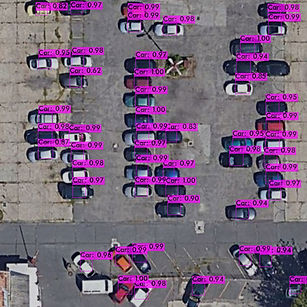top of page

#### SATELLITE CAR & SWIMMINGPOOL DETECTOR (YOLOV4)On this website you can try the model with your own images and buy it if you are satisfied with its accuracy.

Model description:

This model detects vehicles and swimming pools in images taken from above (by drone or satellite). The model expects an image of an area of about 50 square meters as input.

Number of classes: 2 (car; swimmingpool)

Metrics:

detections_count = 9889, unique_truth_count = 3301
class_id = 0, name = Car, ap = 82.90%            (TP = 2225, FP = 293)
class_id = 1, name = Swimmingpool, ap = 83.00%           (TP = 507, FP = 87)

for conf_thresh = 0.25, precision = 0.88, recall = 0.83, F1-score = 0.85
for conf_thresh = 0.25, TP = 2732, FP = 380, FN = 569, average IoU = 68.23 %

IoU threshold = 50 %, used Area-Under-Curve for each unique Recall
mean average precision (mAP@0.50) = 0.829488, or 82.95 %

System requirements:

Inference time using CPU: 300 ms (on HP Laptop 15-DA0042NH (Processor: Intel(R) Core(TM) i7-8550U CPU))

bottom of page